# Semi-hyperbolic space

(diff) ← Older revision | Latest revision (diff) | Newer revision → (diff)

A projective-space in which the metric is defined by a given absolute consisting of the following collection: a second-order real cone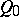of index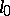with an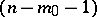-plane vertex; a real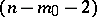-coneof index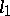with an-plane vertex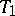in the-plane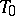;; a real-cone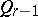of index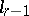with an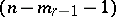-plane vertex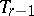; and a non-degenerate real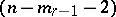-quadric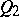of indexin the plane;. This is the definition of a semi-hyperbolic space with indices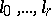; it is denoted by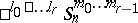.

If the coneis a pair of merging planes, both identical with(for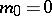), the semi-hyperbolic plane with the improper planeis called a semi-Euclidean space: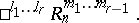The distance between two pointsandis defined as a function of the position of the straight linerelative to the planes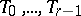. In particular, if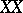does not intersect, the distance betweenandis defined through a scalar product, in analogy with the appropriate definition in a quasi-hyperbolic space. If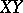intersectsbut does not intersect, or it intersects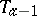but does not intersect, the distance between the points is defined as the scalar product with itself of the distance between the vectors of the pointsand.

Depending on the position of the absolute relative to the planesone distinguishes four types of straight lines of different orders: elliptic, hyperbolic, isotropic, and parabolic.

The angles between the planes in a semi-hyperbolic space are defined analogous to the angles between the planes in a quasi-hyperbolic space, i.e. using distance in the dual space.

A projective metric in a semi-hyperbolic space is a metric of the most general form. A particular case of such a metric is a metric of a quasi-hyperbolic space. In particular, the-planeis identical with the pseudo-Euclidean space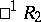, the plane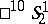— with the co-pseudo-Euclidean space; the-spaces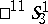and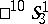coincide with the quasi-hyperbolic-space, the-space— with the co-pseudo-Euclidean space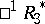, etc. The-space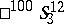is dual to the pseudo-Galilean space, it is known as a co-pseudo-Galilean space; its absolute consists of pairs of real planes (a cone) and a pointon the straight linein which these planes intersect.

The motions of a semi-hyperbolic space are defined as collineations of the space which map the absolute into itself. If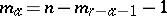and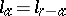, a semi-hyperbolic space is dual to itself. It is then possible to define co-motions, the definition being analogous to that of co-motions in a self-dual quasi-hyperbolic space. The group of motions and the group of motions and co-motions are Lie groups. The motions (and co-motions) of a semi-hyperbolic space are described by pseudo-orthogonal operators with indices determined by the indices of the space.

A semi-hyperbolic space is a semi-Riemannian space.

How to Cite This Entry:
Semi-hyperbolic space. Encyclopedia of Mathematics. URL: http://encyclopediaofmath.org/index.php?title=Semi-hyperbolic_space&oldid=18775
This article was adapted from an original article by L.A. Sidorov (originator), which appeared in Encyclopedia of Mathematics - ISBN 1402006098. See original article